Courses

# Polar plots Notes | EduRev

## Electrical Engineering (EE): Polar plots Notes | EduRev

The document Polar plots Notes | EduRev is a part of the Electrical Engineering (EE) Course Control Systems.
All you need of Electrical Engineering (EE) at this link: Electrical Engineering (EE)

Polar Plot

The polar plot of a sinusoidal transfer function G(jω) is a plot of the magnitude of G(jω) versus the phase angle of G(jω) on polar coordinates as ω varied from zero to infinity.

Procedure to Sketch the Polar Plot

• Step-1: Determine transfer function G(jω).
• Step-2: Put s = jω in the transfer function to obtain G(jω).
• Step-3: At ω = 0 and m = ∞, calculate |G(jω)| by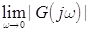and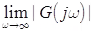. .
• Step-4: Calculate the phase angle of G(jω) at ω = 0 and ω = ∞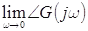and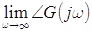.
• Step-5: Rationalize the function G(jω) and separate the real and imaginary axis.
• Step-6: Equate the imaginary part Im|G(jω)| to zero and determine the frequencies at which plot intersects the real axis and calculate the value of G(jω) at the point of intersection by substituting the determined value of frequency in the expression of G(jω).
• Step-7: Equate the real part Re|G(jω)| to zero and determine the frequencies at which plots intersects the imaginary axis and calculate the value G(jω) at the point of intersection by substituting the determined value of frequency in the rationalized expression of G(jω).
• Step-8: Sketch the polar plot with the help of above information.

Polar Plot of Some Standard Functions

• Type 0 System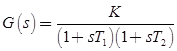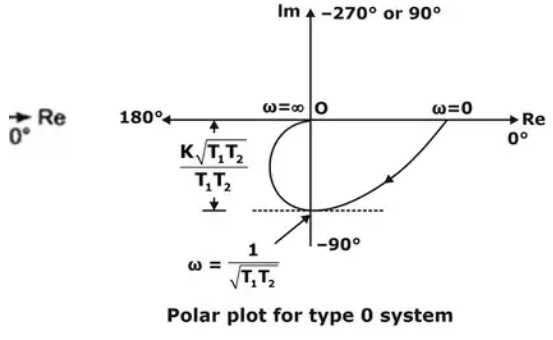• Type 1 System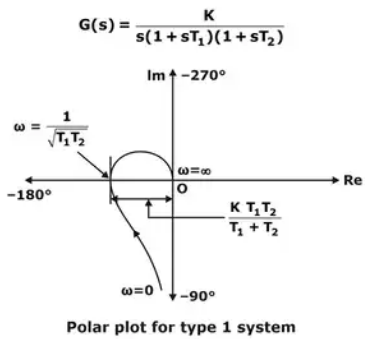• Type 2 System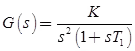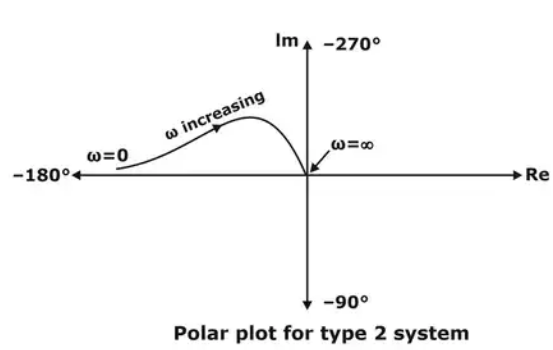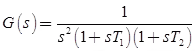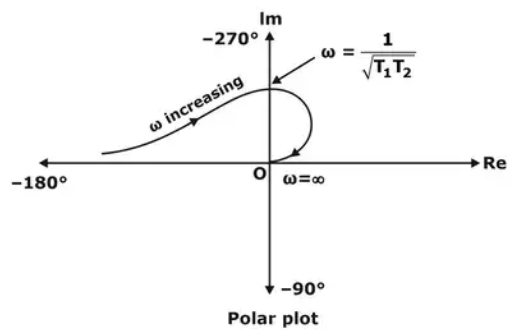Polar Plot for 1 / s = G(s)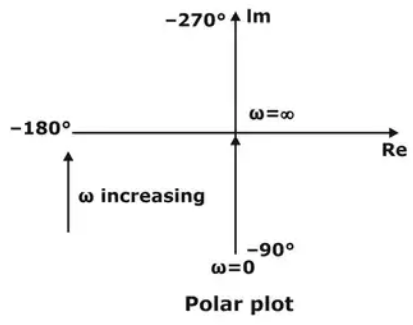Polar Plot For  1 / s2 = G(s)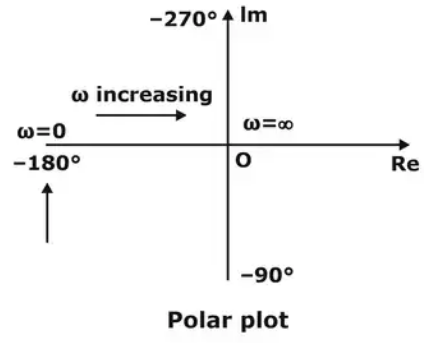Gain Margin and Phase Margin with Polar Plot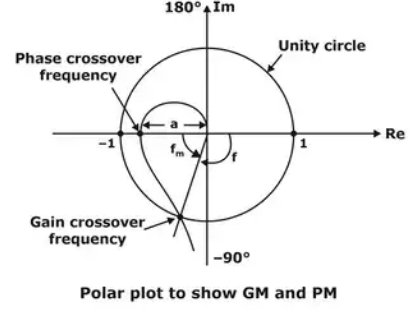• Phase Crossover Frequency: The frequency at which the polar plot crosses the -180° line is called phase crossover frequency.
• Gain Crossover Frequency: The frequency at which the polar plot crosses the unity circle is called gain crossover frequency.
• Gain Margin: At phase crossover frequency, if gain is 'a' then
Gain margin = - 20 log a
• Phase Margin (φm): At gain crossover frequency, if phase is φ then phase margin φm = 180 + φ
where, φ is positive from anti-clockwise direction.

Nyquist Stability Criterion

A stability test for time invariant linear systems can also be derived in the frequency domain. It is known as Nyquist stability criterion. It is based on the complex analysis result known as Cauchy’s principle of argument. Nyquist criterion is used to identify the presence of roots of a characteristic equation of a control system in a specified region of s-plane. Nyquist approach is same as Routh-Hurwitz but, it differ with following aspect

• The open loop transfer function "G(s)H(s)" is considered instead of closed loop characteristic equation "1 + G(s) H(s) = 0".
• Inspection of graphical plot of G(s) H(s) enables to get more than yes or no answer of Routh-Hurwitz method pertaining to stability of control system.
• Nyquist plots display both amplitude and phase angle on a single plot, using frequency as a parameter in the plot.
• Nyquist plots have properties that allow you to see whether a system is stable or unstable. It will take some mathematical development to see that, but it's the most useful property of Nyquist plots.

Concept of Encirclement & Enclosement

1. Encirclement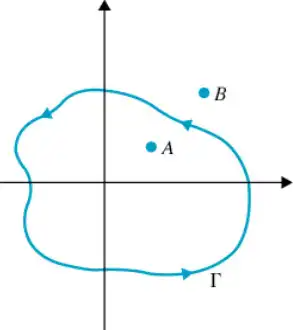• point A is encircled in the counter in Counter Clock Wise by closed path, while point B is NOT encircled by closed path.

2. Enclosement: A point or region is said to be enclosed by a closed path if the point or region lies to the right of the path when the path is traversed in any prescribed direction.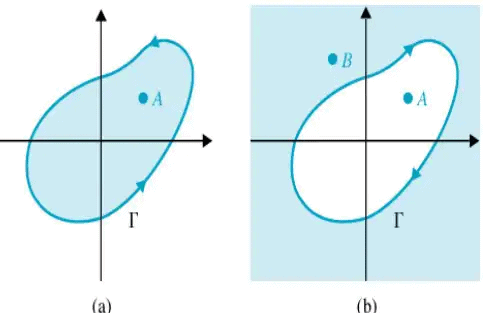• In figure (a) point A is not enclosed by Path, while Point B is enclosed by path.
• In figure (b) point A is enclosed by Path, while point B is not enclosed by path.

Nyquist Stability Criterion: Fundamentals
Consider the phasor from point A to S1 of encirclement = N, the net angle traversed by the phasor = 2πN rad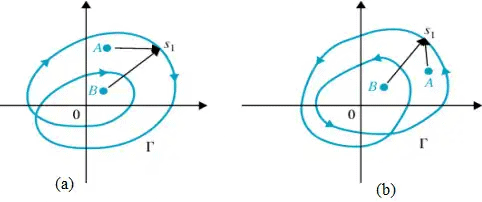• In figure (a) Point A of encirclement = +1; Point B of encirclement = +2.
• In figure (b) Point A of encirclement = -1; Point B of encirclement = -2.

Determination of N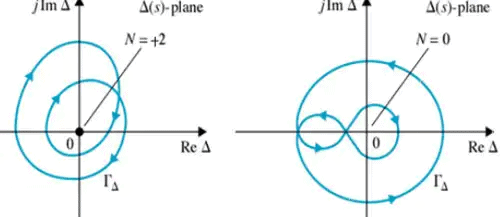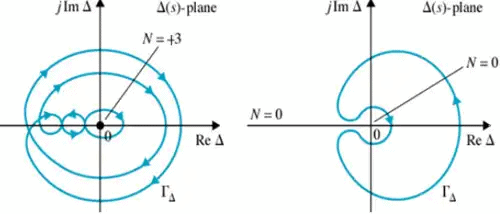• For a SISO feedback system the closed-loop transfer function is given by
M(s) = G(s) / 1+ H(s)G(s)
• closed-loop system poles are obtained by solving the following equation
1 + G(s)H(s) = 0 = Δ(s) represents the system characteristic equation.
• In the following we consider the complex function
D(s) = 1 + G(s)H(s)
• The zeros of D(s) are the closed-loop poles of the transfer function. Here now we concluded that the poles of D(s) are the zeros of M(s).
• At the same time the poles of D(s) are the open-loop control system poles since they are contributed by the poles of H(s)G(s), which can be considered as the open-loop control system transfer function—obtained when the feedback loop is open at some point.
• The Nyquist stability test is obtained by applying the Cauchy principle of argument to the complex function D(s). First, we state Cauchy’s principle of argument.

Cauchy’s Principle of Argument

• Let F(s) be an analytic function in a closed region of the complex s-plane, except at a finite number of points (namely, the poles of F(s)).
• It is also assumed that F(s) is analytic at every point on the contour. Then, as 's' travels around the contour in the s-plane in the clockwise direction, the function F(s) encircles the origin in the [ReF{(s)}, Img{F(s)}]-plane in the same direction N times, where N given By
N = P - Z
where Z and P stand for the number of zeros and poles (including their multiplicities) of the function F(s) inside the contour.
• The above result can be also written as
arg{F(s)} = (Z - P)2π = 2Nπ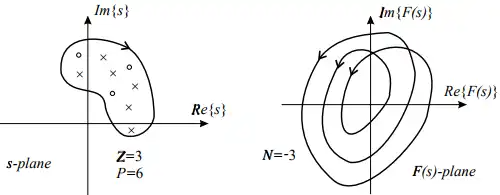Nyquist Plot
The Nyquist plot is a polar plot of the function D(s) = 1 + G(s)H(s) when travels around the contour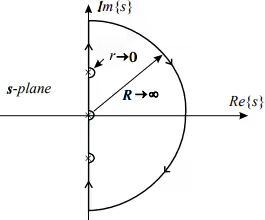• The contour in the above figure covers the whole unstable half plane of the complex plane s, R → ∞.
• Since the function D(s), according to Cauchy’s principle of argument, must be analytic at every point on the contour, the poles D(s) of on the imaginary axis must be encircled by infinitesimally small semicircles.

Nyquist Criterion

• It states that the number of unstable closed-loop poles is equal to the number of unstable open-loop poles plus the number of encirclements of the origin of the Nyquist plot of the complex function F(s).
• The above criterion can be slightly simplified if instead of plotting the function "D(s) = 1 + G(s)H(s)", we plot only the function G(s)H(s) and count encirclement of the Nyquist plot of G(s)H(s) around the point (-1 + j0)
• The number of unstable closed-loop poles (Z) is equal to the number of unstable open-loop poles (P) plus the number of encirclements (N) of the point (-1 + j0) of the Nyquist plot of G(s)H(s), that is
Z = P + N

Phase and Gain Stability Margins
Two important notions can be derived from the Nyquist diagram: phase and gain stability margins. The phase and gain stability margins are presented in Figure below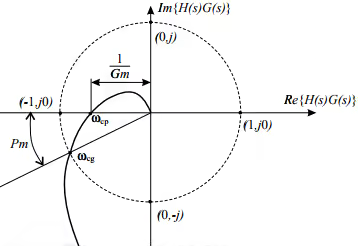• They give the degree of relative stability; in other words, they tell how far the given system is from the instability region. Their formal definitions are given by

Pm = 180° + arg{G(jωcg)H(jωcg)}
GM[dB] = 20 log(1/|G(jωcp)H(jωcp)|) [dB]

• where ωgc and ωpc stand for, respectively, the gain and phase crossover frequencies, which are obtained from the figure as
|G(jωcg)H(jωcg)| = 1 ⇒ ωcg
arg {G(jωcp)H(jωcp)} = 180°⇒ωcp
Example: Consider a control system represented by
G(s)H(s) = 1/(s(s+1))
Solution: Since this system has a pole at the origin, the contour in the -plane should encircle it with a semicircle of an infinitesimally small radius. This contour has three parts (a), (b), and (c). Mappings for each of them are considered below.
• On this semicircle the complex variable 's' is represented in the polar form by 's = Re' with R→∞, - π/2 ≤ ψ ≥ π/2. Substituting 's = Re' into G(s)H(s), we easily see that G(s)H(s)→0 . Thus, the huge semicircle from the -plane maps into the origin in the -plane .
Thus, the huge semicircle from the s-plane maps into the origin in the G(s)H(s)-plane.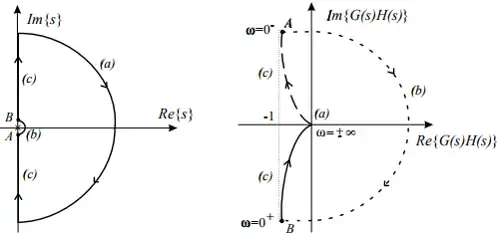• On this semicircle the complex variable is represented in the polar form by 's = re' with r→0, - π/2 ≤ ψ ≥ π/2. so that we have
G(s)H(s) → 1/re → ∞ x arg(-φ)
Since φ changes from  '- π/2' at point A to ' π/2' at point B, arg{G(s)H(s)} will change from "π/2 to -π/2"  . We conclude that the infinitesimally small semicircle at the origin in the s-plane is mapped into a semicircle of infinite radius in the G(s)H(s)-plane.
• On this part of the contour s takes pure imaginary values, i.e.  s = jω with ω  changing  from'-∞ to +∞'.Due to symmetry, it is sufficient to study only mapping along "0 + ≤ ω ≥ + ∞" . We can find the real and imaginary parts of the function G(jω)H(jω), which are given by
Re{G(jω)H(jω)} = -1/ω+ 1
Im{G(jω)H(jω)} = -1/ω(ω+ 1)

From the above expressions, we see that neither the real nor the imaginary parts can be made zero, and hence the Nyquist plot has no points of intersection with the coordinate axis. For ω = 0+ we are at point B and since the plot at  ω = +∞ will end up at the origin. Note that the vertical asymptote of the Nyquist plot is given by
{Re G(jo±)H(jo±)} = -1
• From the Nyquist diagram we see that N = 0 and since there are no open-loop poles in the left half of the complex plane, i .e. P = 0, we have Z = 0 so that the corresponding closed-loop system has no unstable poles.
The document Polar plots Notes | EduRev is a part of the Electrical Engineering (EE) Course Control Systems.
All you need of Electrical Engineering (EE) at this link: Electrical Engineering (EE)Use Code STAYHOME200 and get INR 200 additional OFF Use Coupon Code
All Tests, Videos & Notes of Electrical Engineering (EE): Electrical Engineering (EE)

### Top Courses for Electrical Engineering (EE)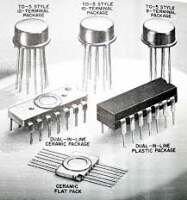## Control Systems

8 docs|30 tests

### Top Courses for Electrical Engineering (EE)Track your progress, build streaks, highlight & save important lessons and more!

,

,

,

,

,

,

,

,

,

,

,

,

,

,

,

,

,

,

,

,

,

;